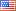# Rheological articles

## Flow Curve Behavior of Polymer Melts

low curves of polymer melts (the graph of viscosity versus shear rate in the log-log scale) has two distinct behavior of Newtonian and Power law.  At very low shear rates the Newtonian behavior dominates where the viscosity is independent of the shear rate and it approaches the value of  which has been named as the zero-shear viscosity. After that, the viscosity begins to reduce at some critical shear rate (y0). At higher shear rates, the Power law behavior dominates where the viscosity decreases with increasing shear rate with negative slope of (n - 1).

#### 11.15.2019

Flow curves of polymer melts (the graph of viscosity versus shear rate in the log-log scale) has two distinct behavior of Newtonian and Power law.  At very low shear rates the Newtonian behavior dominates where the viscosity is independent of the shear rate and it approaches the value of  which has been named as the zero-shear viscosity. After that, the viscosity begins to reduce at some critical shear rate (y0). At higher shear rates, the Power law behavior dominates where the viscosity decreases with increasing shear rate with negative slope of (n - 1). The  parameter has been named as Power-law index.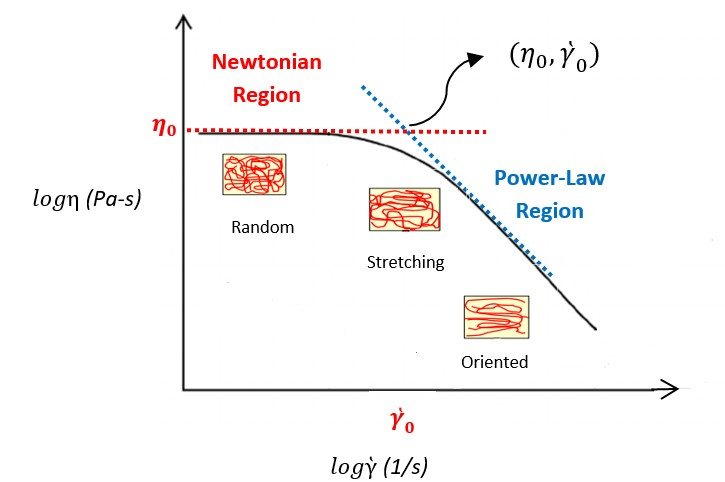Figure 1: Typical flow curve of polymer melts

As it can be seen in Figure 1, the flow curve of polymer melts is bound by two limiting sloes (dashed lines) which determine the zero-shear viscosity value (n00  and the Power-Law index value (n). These parameters can be used to estimate molecular weight (Mw)  and the breadth of molecular weight distribution (MWD). Temperature, pressure, plasticizer, filler, and lubricant are other factors which may have effect on the detailed shape of the viscosity-shear rate curve.

Few quantitative relationships have been defined in order to represent the shear rate dependency of viscosity of polymer materials such as Power-Law model, Bird-Carreau-Yasuda model, Cross model, and Cross-WLF model. Power-law model has been represented as equation below: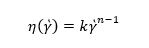where k is consistency (Pa-s) and n is Power-law index. Typically, polymer melts have n value in the range of 0.2-0.6 depending on type of the polymer, its chemical structure, , and . Power-law model only fits the shear-thinning (Power law) portion of the curve and it is a useful model for process control. However, it cannot describe the Newtonian region of the curve. Normally, the capillary rheometer data fall into the shear-thinning region of the curve and can be perfectly fitted by Power-law model. Figure 2 shows the Power-law model fitting analysis (from LabKars software) on two types of polyethylene (PE) materials using a Dynisco LCR7001 capillary rheometer.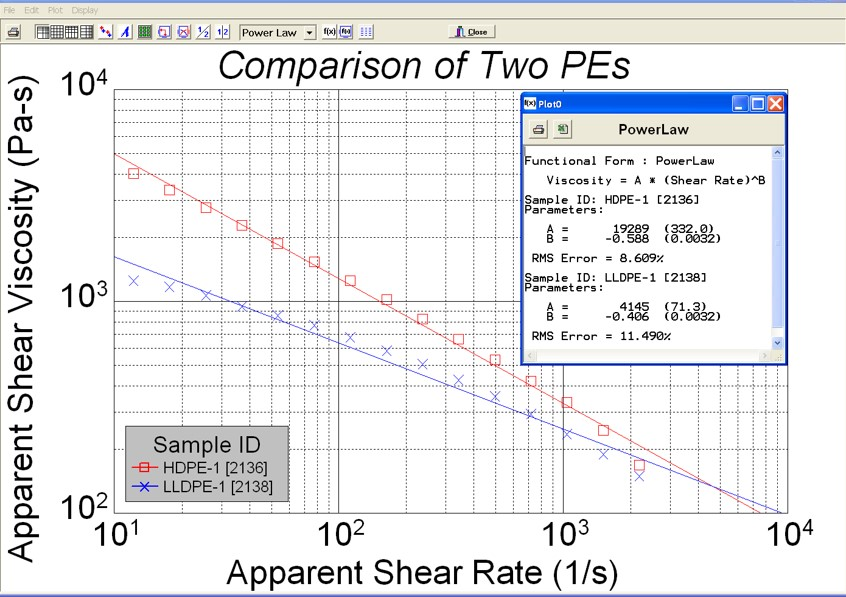Figure 2. Power-law model fitting analysis

Cross model has been represented as equation below: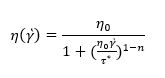where  is the shear viscosity (Pa-s), t is the critical shear stress (Pa) or stress at the break in the curve, and  is Power-law index. Figure 3 shows the modified Cross model fitting analysis (from LabKars software) on two types of PE materials using a Dynisco LCR7001 capillary rheometer.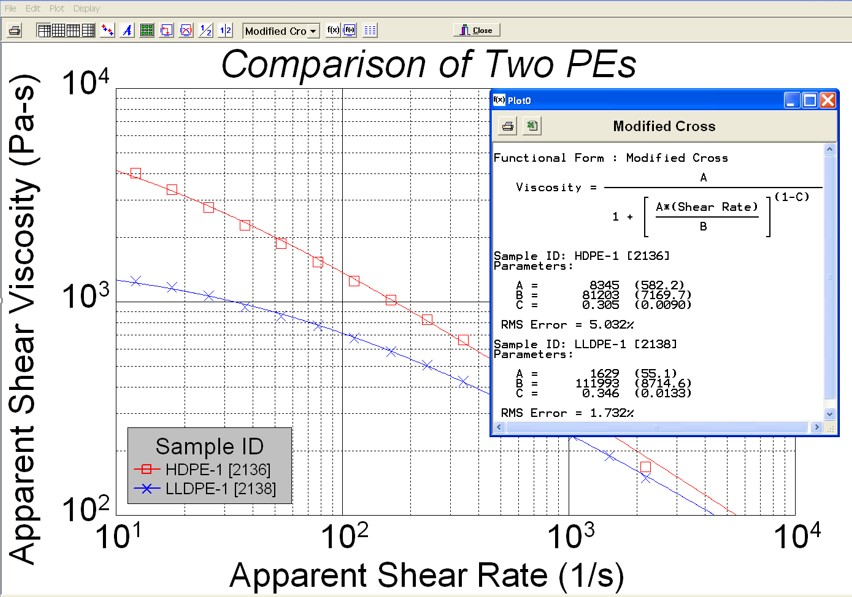Figure 3. Modified Cross model fitting analysis

This model combines the Power-law and Newtonian regions and represents a more complete description of the flow curve. It is a useful model for capturing the zero-shear viscosity value.

References

• J.M. Dealy, and K.F. Wissbrun, Melt Rheology and its Role in Plastics Processing: Theory and Applications, Van Nostrand Reinhold, New York (1990).
• C.L. Rohn, Analytical Polymer Rheology, Hanser (1995).